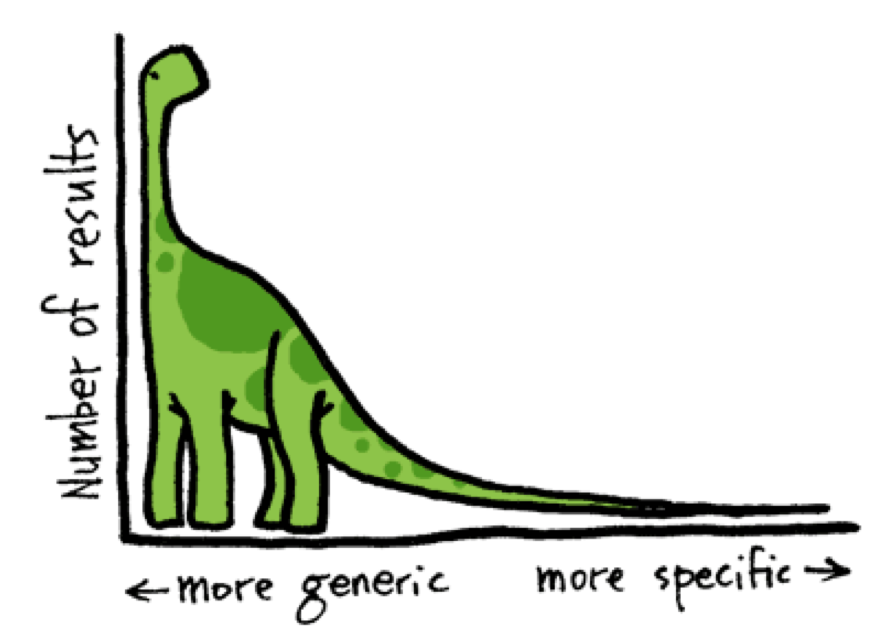# 长尾分布的多标签文本分类平衡方法

## November 19, 2021 • Read: 4281 • Deep Learning • 阅读设置EMNLP2021上有一篇名为Balancing Methods for Multi-label Text Classification with Long-Tailed Class Distribution的论文详细探讨了各种平衡损失函数对于多标签分类问题的效果，从最初的BCE Loss到Focal Loss等，感觉这篇文章更像是平衡损失函数的综述。源码在Roche/BalancedLossNLP

### Loss Functions

$$\mathcal{L}_{\text{BCE}} = \begin{cases}-\log (p_i^k)\quad &\text{if } y_i^k =1\\-\log (1-p^k_i)\quad &\text{otherwise} \end{cases}$$

#### Focal Loss (FL)

$$\mathcal{L}_{\text{FL}} = \begin{cases} -(1-p_i^k)^\gamma \log (p_i^k)\quad &\text{if } y_i^k =1\\ -(p_i^k)^\gamma \log (1-p_i^k)\quad &\text{otherwise} \end{cases}$$

#### Class-balanced focal loss (CB)

$$r_{\text{CB}} = \frac{1-\beta}{1-\beta^{n_i}}$$

$$\mathcal{L}_{\text{CB}} = \begin{cases} -r_{\text{CB}} (1-p_i^k)^\gamma \log (p_i^k) \quad &\text{if } y_i^k =1\\ -r_{\text{CB}} (p_i^k)^\gamma \log (1-p_i^k) \quad &\text{otherwise} \end{cases}$$

#### Distribution-balanced loss (DB)

$$r_{\text{DB}} = P_i^C / P^I$$

$$\hat{r}_{\text{DB}} = \alpha + \sigma(\beta \times (r_{\text{DB}} - \mu))$$

$$\mathcal{L}_{\text{R-FL}} = \begin{cases} -\hat{r}_{\text{DB}} (1-p_i^k)\log (p^k_i) \quad &\text{if } y_i^k =1\\ -\hat{r}_{\text{DB}} (p_i^k)\log (1-p^k_i) \quad &\text{otherwise} \end{cases}$$

$$\mathcal{L}_{\text{NTR-FL}} = \begin{cases} - (1-q_i^k)\log (q^k_i) \quad &\text{if } y_i^k =1\\ -\frac{1}{\lambda} (q_i^k)\log (1-q^k_i) \quad &\text{otherwise} \end{cases}$$

$$\hat{b}_i = -\log (\frac{1}{p_i} - 1), \ v_i = -\kappa \times \hat{b}_i$$

$$\mathcal{L}_{\text{{DB}}} = \begin{cases} - \hat{r}_{\text{DB}}(1-q_i^k)\log (q^k_i) \quad &\text{if } y_i^k =1\\ -\hat{r}_{\text{DB}}\frac{1}{\lambda} (q_i^k)\log (1-q^k_i) \quad &\text{otherwise} \end{cases}$$

### Result### 个人总结